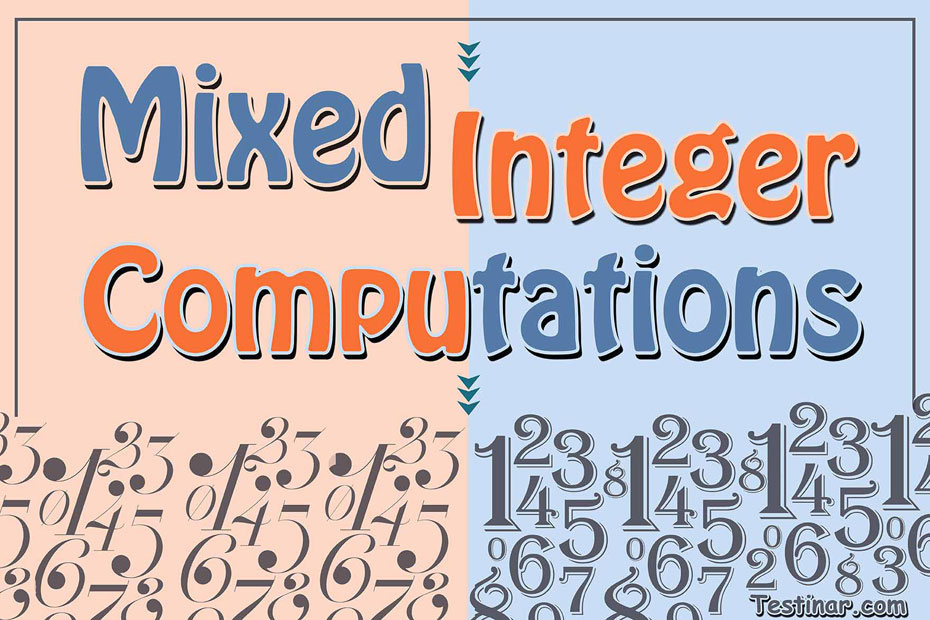## How to Do Mixed Integer Computations

The term integers represent the meaning “intact” or “whole”. So, you can generally refer an integer as a whole number, except integers can be negative also!

What is an Integer?

An integer is a whole number (not any decimal or fraction numbers) which can be zero, positive or negative numbers. Some examples of integers can be $$7 \ , \ 3 \ , \ 0 \ , \ -5 \ , \ -15,$$ etc. Moreover, we can represent integers by the denotation $$Z$$ which comprises of:
Positive Integers: As the name suggests, any integer that is greater than zero is termed as a positive integer.
Negative Integer:  As from the name, any integer that is less than zero is termed as a negative integer.
Zero: Zero is neither a positive integer or a negative integer. It is just a whole number.
So, we can write $$Z = \{….,-5 \ , \ -4 \ , \ -3 \ , \ -2 \ , \ -1 \ , \ 0 \ , \ 1 \ , \ 2 \ , \ 3 \ , \ 4 \ , \ 5,……\}$$
Also, we can place all integers on a number line where the negative ones are placed on the left of $$“0”$$ and the positive ones on the right. Moreover, we can perform the 4 basic mathematic properties with integers. They are:

• Subtraction
• Multiplication
• Division

We often see that negative integers are always written as $$-5 \ , \ -9$$ and so on. But it is not generally considered necessary to write positive integers like $$+5 \ , \ +9$$ and so on. So, when we write just $$5,$$ we mean $$+5$$.
Another thing to note is, that an absolute value of any integer is always positive. So, $$\lvert -6 \rvert=6$$ and $$\lvert 6 \rvert$$  is also $$6$$.

How to Add and Subtract Integers

• In the first case, if two integers have the same sign (either both are positive or both are negative), add up those integers and put the common sign.
• In the second case, if two integers have an opposite sign (one is positive and the other one negative), then subtract them and put the sign of the bigger number.

Example:

• $$-12 +13 = +1,$$
• $$-12 -13 = -25,$$
• $$12 – 13 = -1$$ and so on.

How to Multiply and Divide Integers

To Multiply or Divide two integers, follow these steps:

• Firstly, perform general multiplication or division between the two integers and ignore their sign.
• Next, we have to decide the sign. So, if both signs are opposite, then we must always put a negative sign. Also, if both signs of the integers are same, we must use a positive sign.

Example:

• $$-12 \times +7 = -84,$$
• $$-12 \div +6 = -2,$$
• $$12 \div 3 = 4$$ and so on.

### Exercises for Mixed Integer Computations

1) $$(-4) \ \times \ (-3) \ =$$

2) $$36 \ \div \ (-4) \ =$$

3) $$-14 \ \div \ 2 \ =$$

4) $$36 \ \div \ 6 \ =$$

5) $$-24 \ \div \ (-3) \ =$$

6) $$2 \ \div \ 2 \ =$$

7) $$-56 \ \div \ (-8) \ =$$

8) $$24 \ \div \ (-4) \ =$$

9) $$8 \ \div \ (-1) \ =$$

10) $$4 \ \times \ (-7) \ =$$

1) $$(-4) \ \times \ (-3) \ = \color{red}{12}$$
2) $$36 \ \div \ (-4) \ = \color{red}{-9}$$
3) $$-14 \ \div \ 2 \ = \color{red}{-7}$$
4) $$36 \ \div \ 6 \ = \color{red}{6}$$
5) $$-24 \ \div \ (-3) \ = \color{red}{8}$$
6) $$2 \ \div \ 2 \ = \color{red}{1}$$
7) $$-56 \ \div \ (-8) \ = \color{red}{7}$$
8) $$24 \ \div \ (-4) \ = \color{red}{-6}$$
9) $$8 \ \div \ (-1) \ = \color{red}{-8}$$
10) $$4 \ \times \ (-7) \ = \color{red}{-28}$$

## Mixed Integer Computations Practice Quiz

### The Most Comprehensive Praxis Core Math Preparation Bundle

$76.99$36.99

### ACT Math Practice Workbook 2022

$25.99$13.99

### ASVAB Math for Beginners 2022

$44.99$24.99

### GED Math in 10 Days

$26.99$13.99